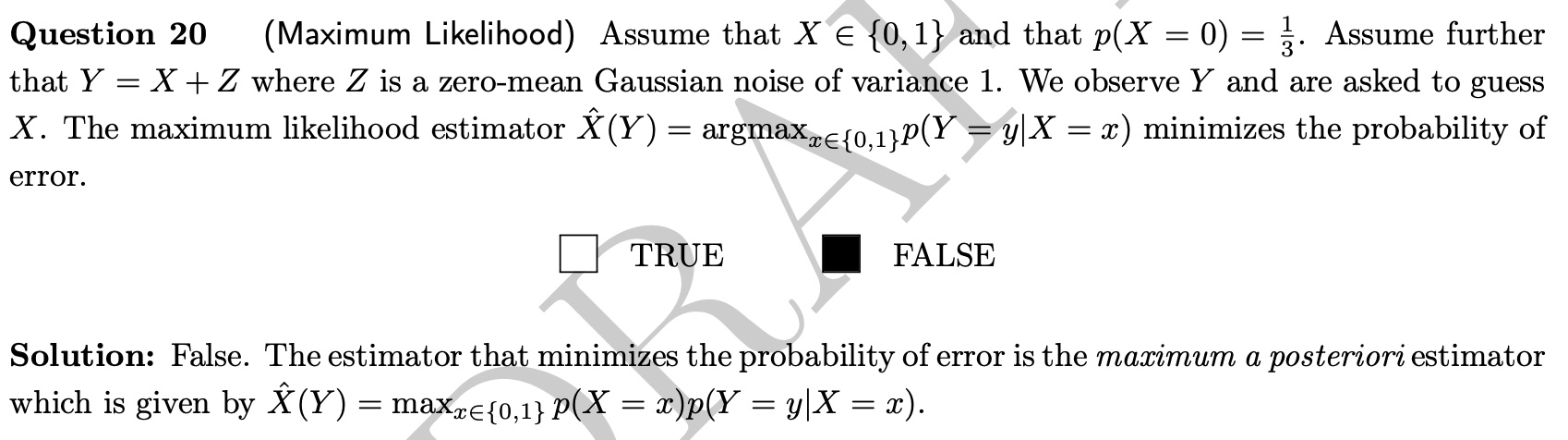### exam 2019 Q20

Hello,
I have a hard time understanding why this is wrong. What would the MLE be in that case or how does the question need to be formulated such that it is true for the MLE?
Thank you for your answerTop comment

The maximum likelihood estimator is correctly given in the question, but it does not minimise the probability of error. The maximum likelihood estimator purely considers the 'model' Y=X+Z, and estimates X based on this model (maximizing the likelihood). This does not directly translate to 'minimizing the probability of error' if you don't take into account how probable the values of X are.

Page 1 of 1